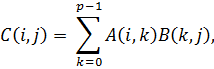ECE 459 - Advanced Computer System Design Laboratory

Experiment 1: MIPS Assembly Language Programming: Matrix Multiplication

Review the help notes for this experiment.

Objectives

The MIPS R2000/R3000 processors were the focus in ECE 451. Therefore, students taking this laboratory course (i.e., ECE 459) have already used the SPIM simulator for the MIPS R2000/R3000 processors in ECE 451. The objective of this experiment is to develop code that uses advanced programming techniques to better understand RISC (Reduced Instruction Set Computer) designs.

Introduction

The matrix product is the most commonly used type of product of matrices. Matrices offer a concise way of representing linear transformations between vector spaces, and matrix multiplication corresponds to the composition of linear transformations. The matrix product of two matrices can be defined when their entries belong to the same ring, and hence can be added and multiplied, and, additionally, the number of the columns of the first matrix matches the number of the rows of the second matrix.

The product of an m × p matrix A with an p × n matrix B is an m × n matrix denoted C whose entries are:where 0 ≤ i ≤ m − 1 is the row index and 0 ≤ j ≤ n − 1 is the column index.

Experiment

Provide a flowchart of your matrix multiplication algorithm. Develop MIPS assembly language code for its implementation. For the sake of simplicity, you may assume that the matrices A, B and C are square matrices, that is, m=n=p. Also, the data type is integer (a 32 bit word in MIPS) and matrices may be stored in memory either row-wise or column-wise. Use the SPIM simulator to execute the code. Show intermediate and final results.